# Ratio of triangles areas

In an equilateral triangle ABC, the point T is its centre of gravity, the point R is the image of the point T in axial symmetry, along the line AB, and the point N is the image of the point T in axial symmetry along the line BC. Find the ratio of the areas of the triangles ABC and TRN.

r =  3:1

### Step-by-step explanation: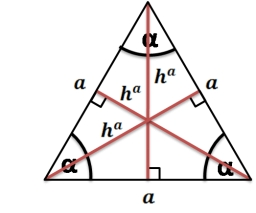Did you find an error or inaccuracy? Feel free to write us. Thank you!Tips to related online calculators
Check out our ratio calculator.
Pythagorean theorem is the base for the right triangle calculator.

#### You need to know the following knowledge to solve this word math problem:

We encourage you to watch this tutorial video on this math problem:

## Related math problems and questions:

• Center of gravityIn the isosceles triangle ABC is the ratio of the lengths of AB and the height to AB 10:12. The arm has a length of 26 cm. If the center of gravity T of triangle ABC find area of triangle ABT.
• MO - trianglesOn the AB and AC sides of the triangle ABC lies successive points E and F, on segment EF lie point D. The EF and BC lines are parallel and is true this ratio FD:DE = AE:EB = 2:1. The area of ABC triangle is 27 hectares and line segments EF, AD, and DB seg
• Equilateral triangle ABCIn the equilateral triangle ABC, K is the center of the AB side, the L point lies on one-third of the BC side near the point C, and the point M lies in the one-third of the side of the AC side closer to the point A. Find what part of the ABC triangle cont
• Infinite sum of areasAbove the height of the equilateral triangle ABC is constructed an equilateral triangle A1, B1, C1, of the height of the equilateral triangle built A2, B2, C2, and so on. The procedure is repeated continuously. What is the total sum of the areas of all tr
• Triangle KLBIt is given equilateral triangle ABC. From point L which is the midpoint of the side BC of the triangle it is drwn perpendicular to the side AB. Intersection of perpendicular and the side AB is point K. How many % of the area of the triangle ABC is area o
• Find the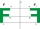Find the image A´ of point A [1,2] in axial symmetry with the axis p: x = -1 + 3t, y = -2 + t (t = are real number)
• Similarity coefficientThe ratio of similarity of two equilateral triangles is 3.5 (ie 7:2). The length of the side of smaller triangle is 2.4 cm. Calculate the perimeter and area of ​​the larger triangle.
• Center of gravity and medianIn the isosceles triangle ABC, the center of gravity T is 2 cm from the base AB. The median parallel to the AB side measures 4 cm. What is the area of the ABC triangle?
• Equilateral triangle v2Equilateral triangle has a perimeter 36 dm. What is its area?
• Construct 8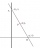Construct an analytical geometry problem where it is asked to find the vertices of a triangle ABC: the vertices of this triangle must be the points A (1,7) B (-5,1) C (5, -11). the said problem should be used the concepts of: distance from a point to a li
• Equilateral triangle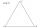Find the area of an equilateral triangle with a side of 15 cm.
• Right trianglesHow many right triangles we can construct from line segments 3,4,5,6,8,10,12,13,15,17 cm long? (Do not forget to the triangle inequality).
• Rectangular trapezoidThe ABCD rectangular trapezoid with the AB and CD bases is divided by the diagonal AC into two equilateral rectangular triangles. The length of the diagonal AC is 62cm. Calculate trapezium area in cm square and calculate how many differs perimeters of the
• Axial sectionCalculate the volume and surface of a cone whose axial section is an equilateral triangle with side length a = 18cm.
• IsoscelesIsosceles trapezium ABCD ABC = 12 angle ABC = 40 ° b=6. Calculate the circumference and area.
• Centre of massThe vertices of triangle ABC are from the line p distances 3 cm, 4 cm and 8 cm. Calculate distance from the center of gravity of the triangle to line p.
• Equilateral triangle vs circle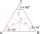Find the area of an equilateral triangle inscribed in a circle of radius r = 9 cm. What percentage of the circle area does it occupy?